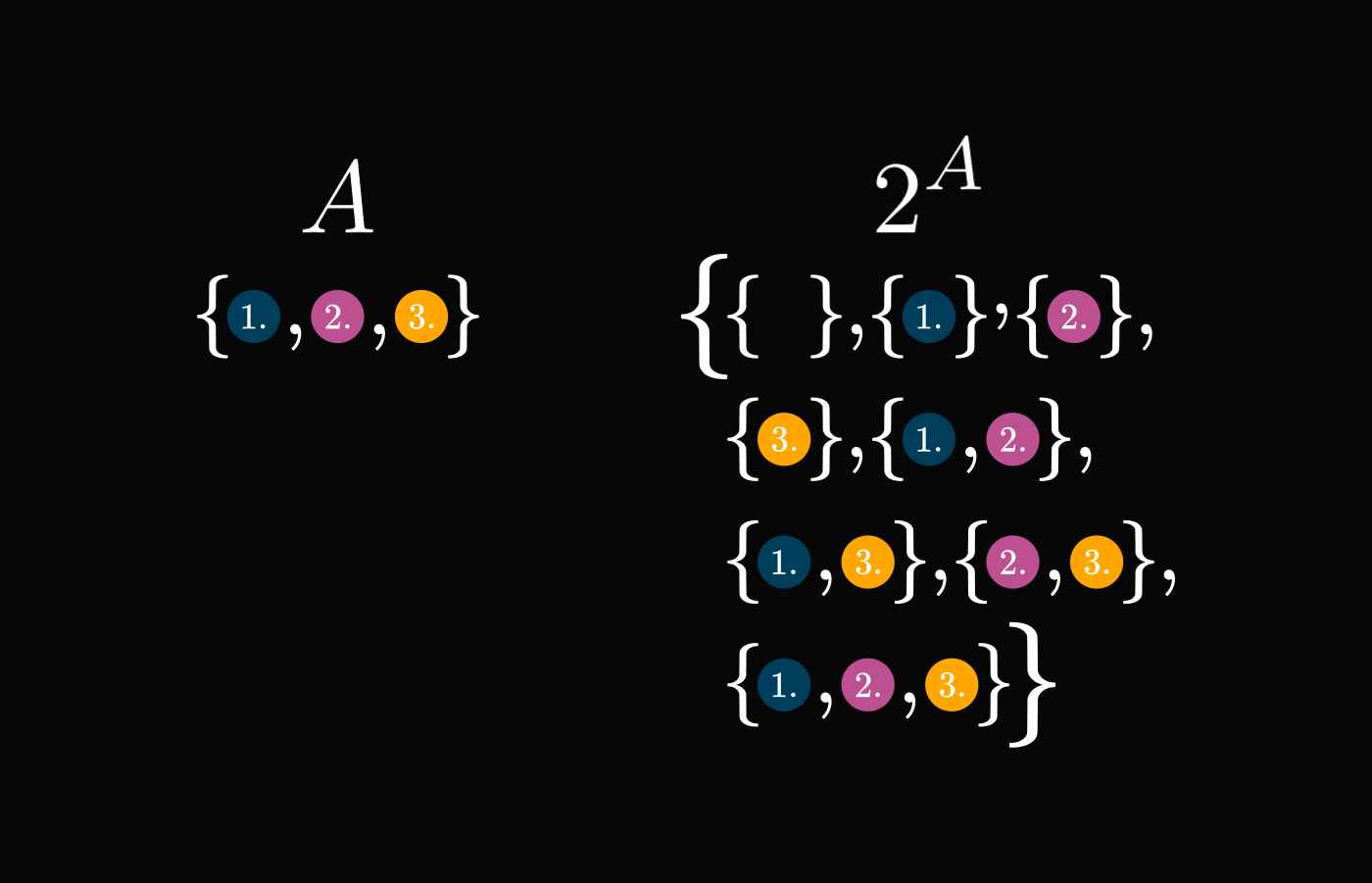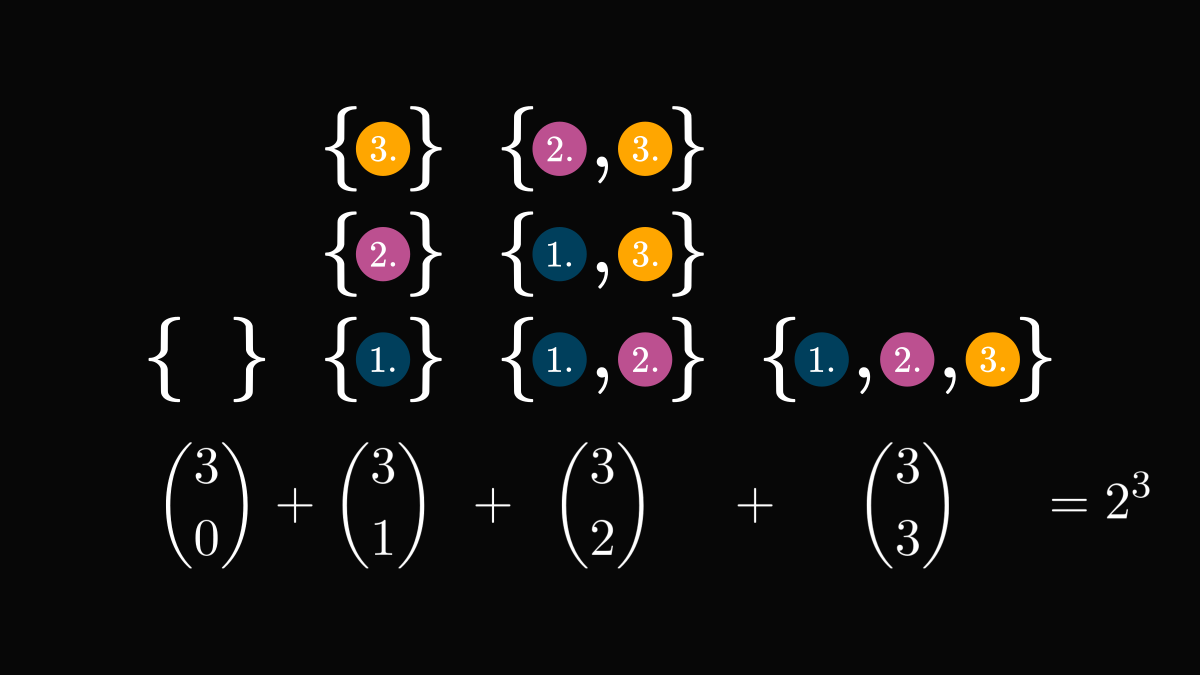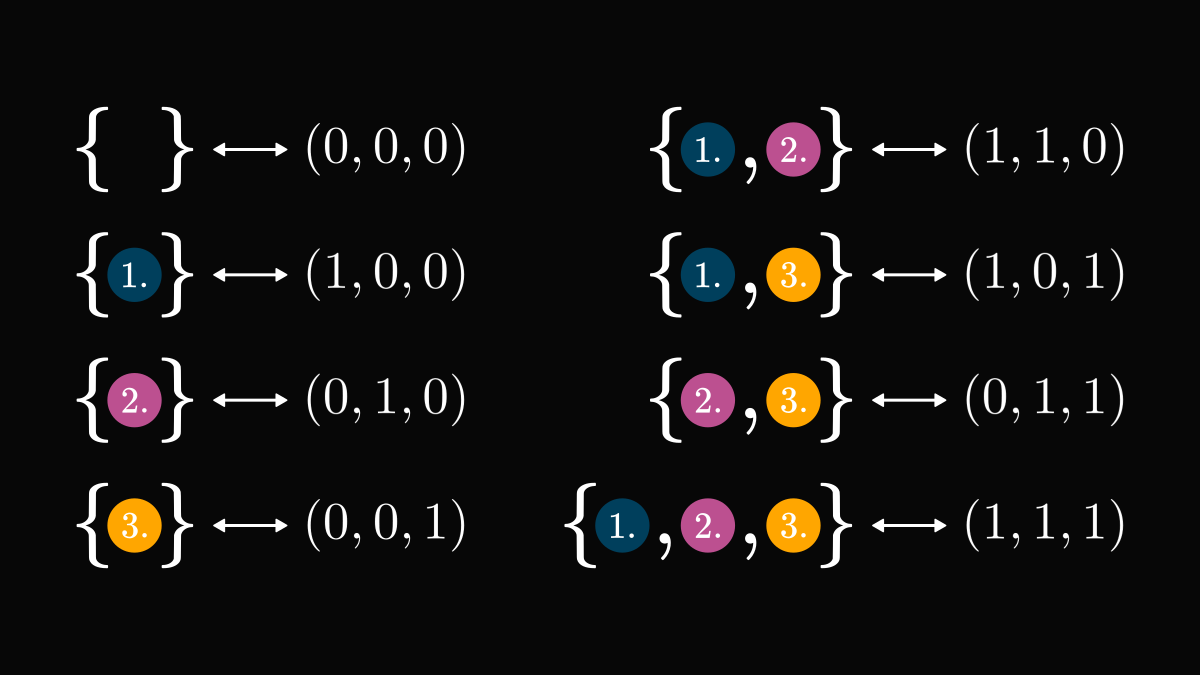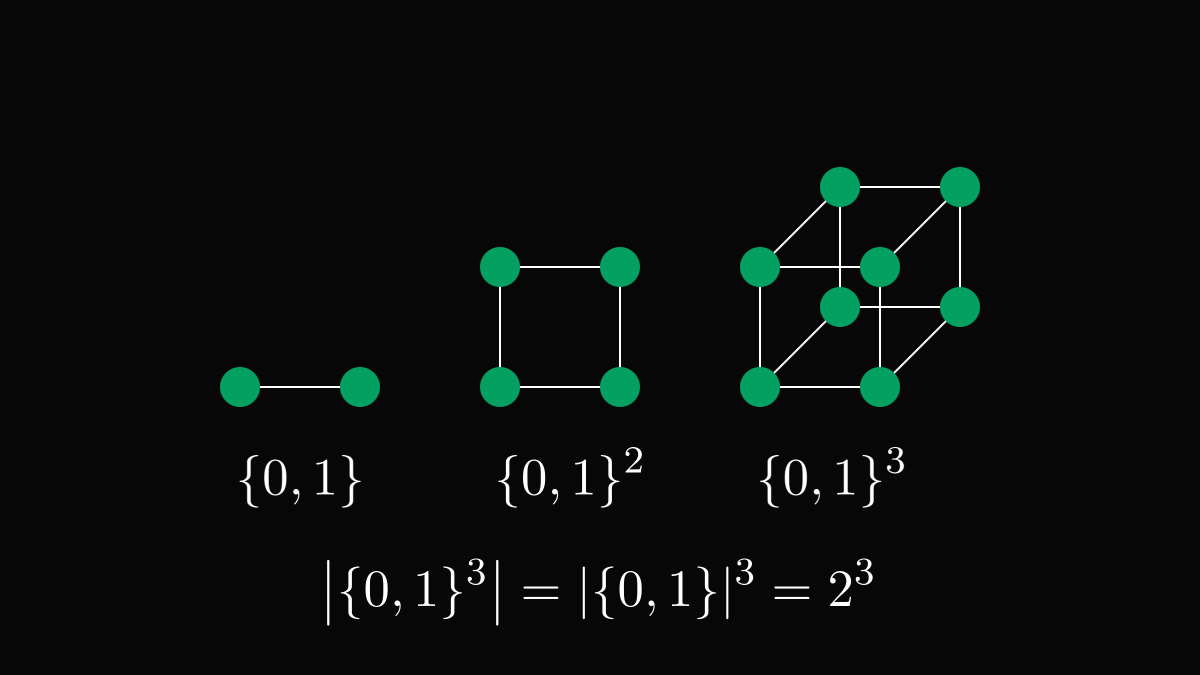# How to count subsetsThere is a profound lesson even in some of the simplest mathematical problems: a good representation is half the success. For example, can you count the number of subsets in a finite set?

Let's see what the solution can teach us!

First things first: notations and definitions. A set between the absolute value symbols denotes the number of elements in the given set. This is called cardinality.

\begin{align*} |A|: &\text{ the number of elements in } A \\ &(\text{the \textit{cardinality} of } A) \end{align*}

Moreover, $\textstyle 2$ raised to the power of a set is just the set of all its subsets. (That is, its power set.)

\begin{align*} 2^A: &\text{ the set of all subsets of } A \\ &(\text{the \textit{power set} of } A) \end{align*}

Back to counting subsets!

A natural idea is to count the subsets of a given size separately and then sum them up. This works as well but requires knowledge about binomial coefficients.However, we can find a much better way by looking at subsets differently!

We can represent each subset with a 0-1 vector, whose components encode the membership of the given elements. This is much easier to count.The membership vectors establish a bijection between the power set and the Cartesian product $\{0, 1\} \times \{0, 1\} \times \{0, 1\}$. Counting the elements of one is the same as counting the other. And elements of Cartesian products are easy to count!

To be more precise, we have the bijection

\begin{align*} f: 2^{\{1, 2, 3\}} &\to \{0, 1\}^3, \\ \{1, 2, 3\} \supseteq S &\mapsto (c_1, c_2, c_3) \in \{0, 1\}^{3}, \end{align*}

where $c_i$ is defined by

$c_i = \begin{cases} 0 & \text{if } i \notin S \\ 1 & \text{if } i \in S. \end{cases}$

By visualizing $\{0, 1\}$ and its powers, we notice a pattern: with each product, the number of elements doubles! Thus, their cardinality is easy to calculate.This method can be applied to any finite set as well. By encoding the subsets by a membership vector, we can establish a bijection between the power set and the powers of $\{0, 1\}$. In this general case, the bijection is defined by

\begin{align*} f: 2^{A} &\to \{0, 1\}^{|A|}, \\ A \supseteq S &\mapsto (c_1, c_2, \dots, c_{|A|}) \in \{0, 1\}^{|A|}, \end{align*}

where $c_i$ is again

$c_i = \begin{cases} 0 & \text{if } i \notin S \\ 1 & \text{if } i \in S. \end{cases}$

Thus, we obtain the general formula describing the cardinality of power sets:

$|2^A| = |\{0, 1\}^{|A|}| = 2^{|A|}.$

You can see for yourself how far a good representation can take us! By utilizing a simple bijection, we transformed the problem into a much simpler one.

In mathematics, this is often the key.